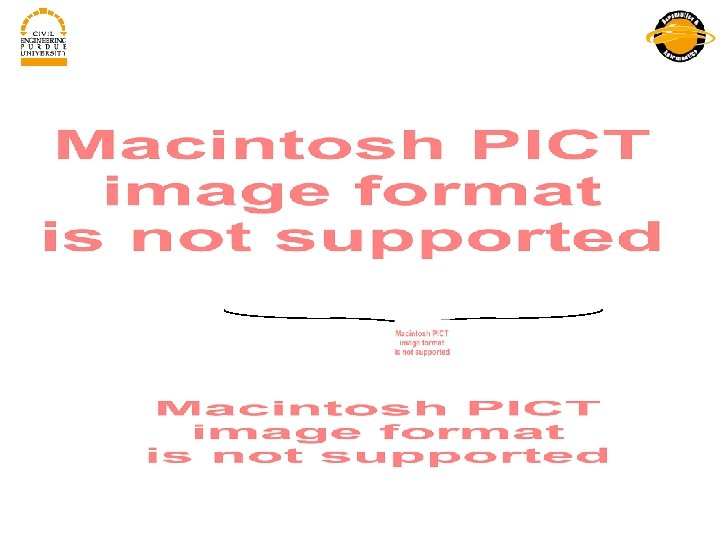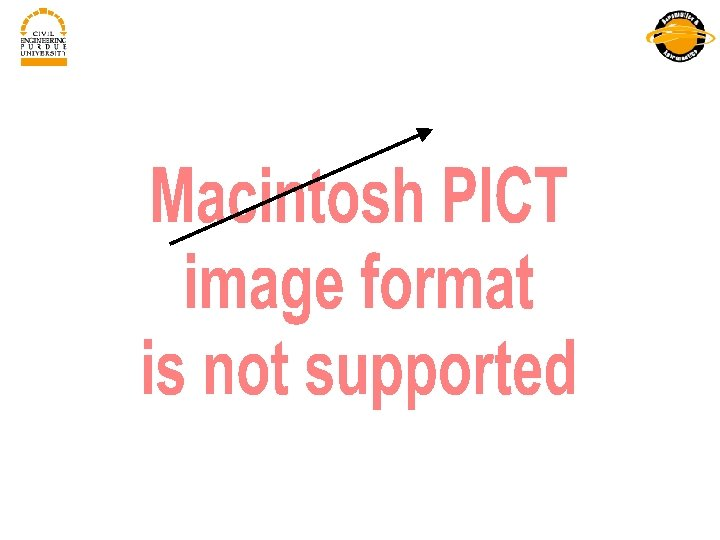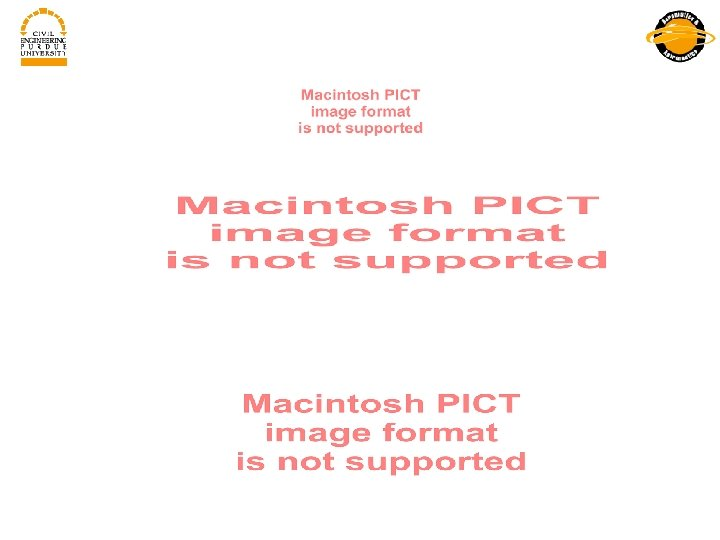Скачать презентацию Outline Overview where

0f45b2f3631b4e9d8e386655baefe4ec.ppt

• Количество слайдов: 31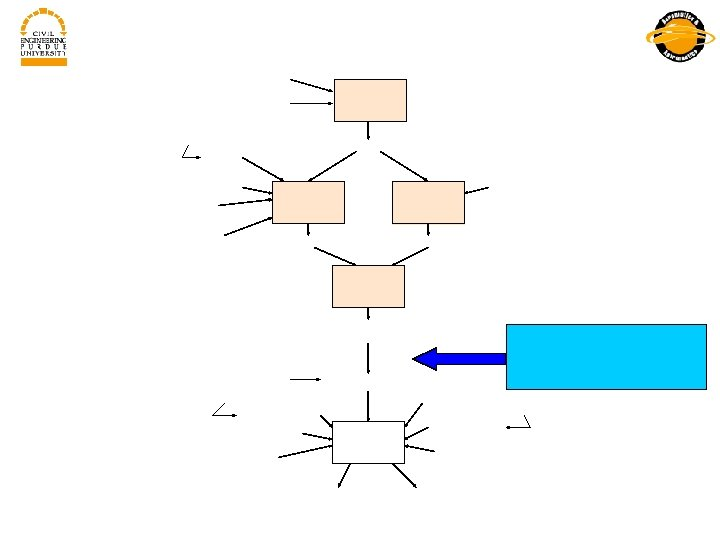Outline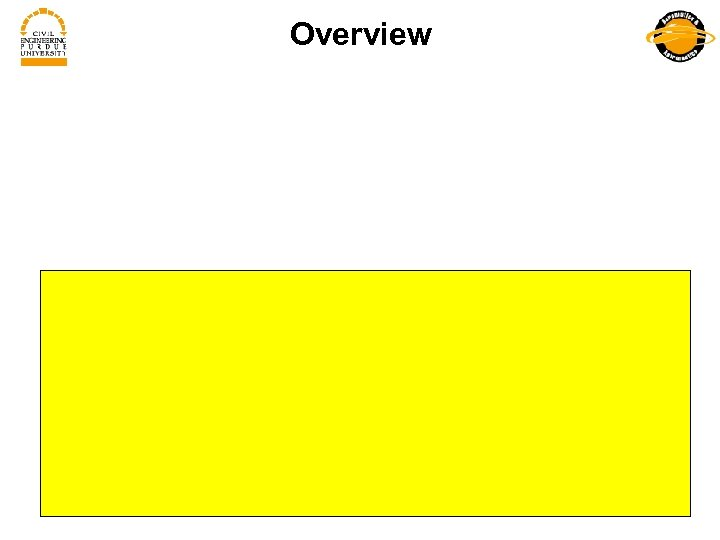Overview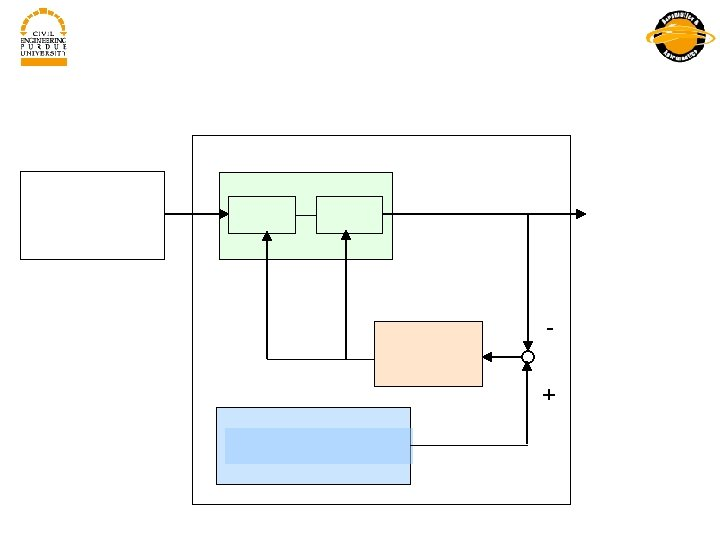+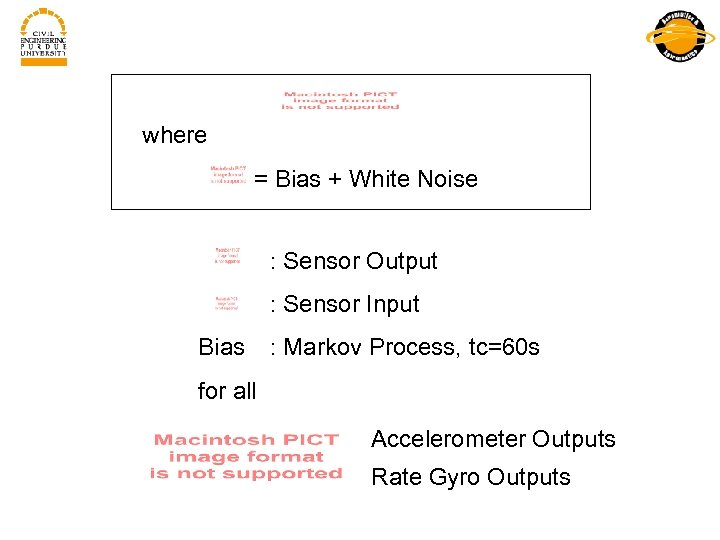where = Bias + White Noise : Sensor Output : Sensor Input Bias : Markov Process, tc=60 s for all Accelerometer Outputs Rate Gyro Outputs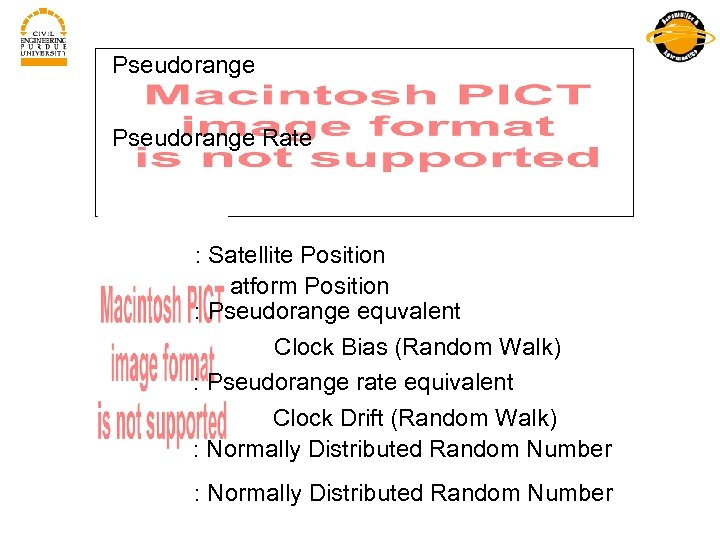Pseudorange Rate : Satellite Position : Platform Position : Pseudorange equvalent Clock Bias (Random Walk) : Pseudorange rate equivalent Clock Drift (Random Walk) : Normally Distributed Random Number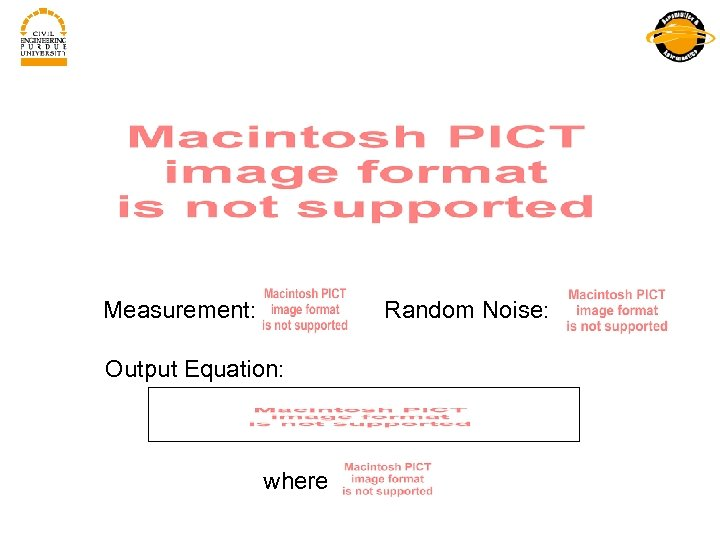Measurement: Random Noise: Output Equation: where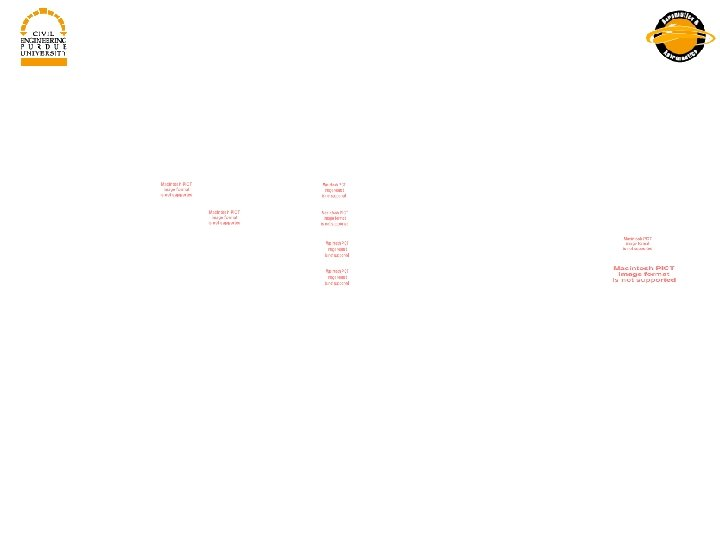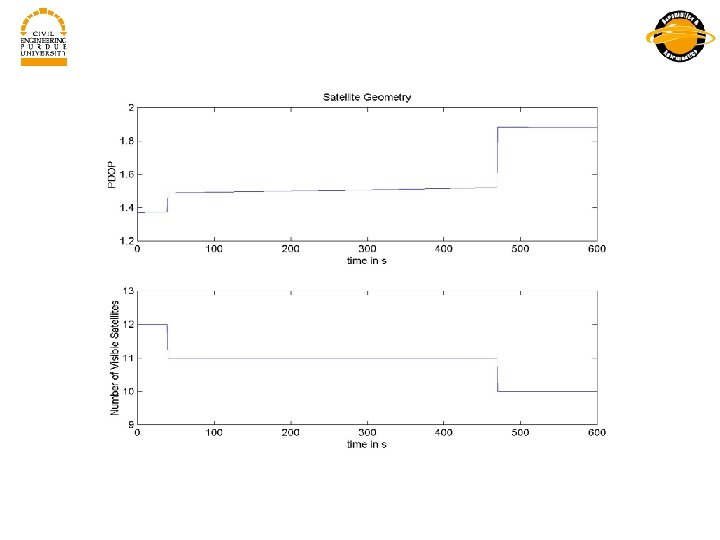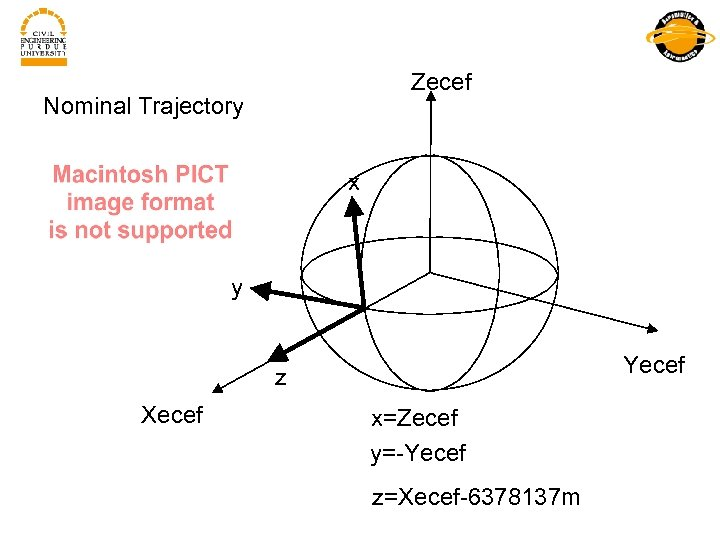Zecef Nominal Trajectory x y Yecef z Xecef x=Zecef y=-Yecef z=Xecef-6378137 m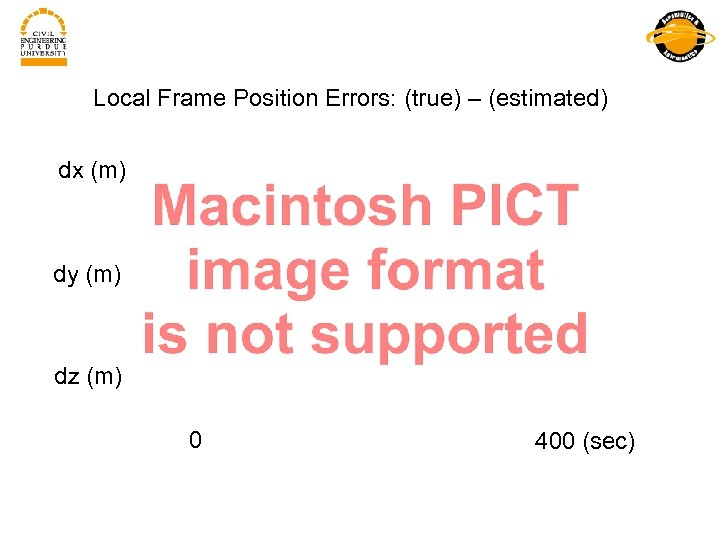Local Frame Position Errors: (true) – (estimated) dx (m) dy (m) dz (m) 0 400 (sec)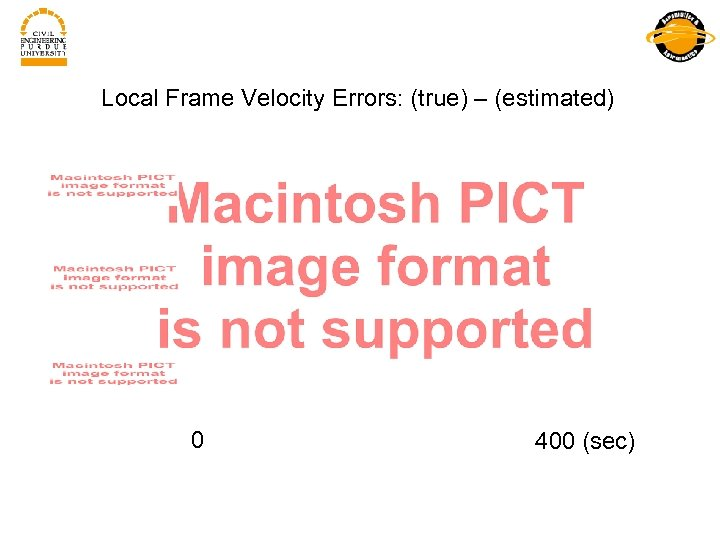Local Frame Velocity Errors: (true) – (estimated) 0 400 (sec)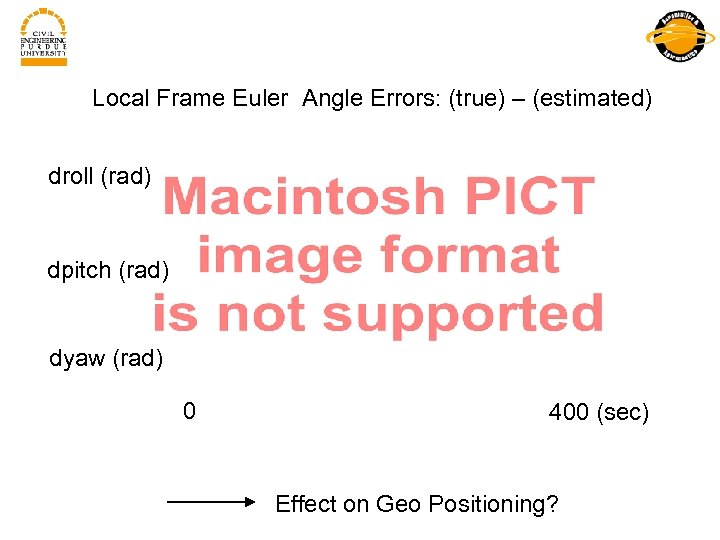Local Frame Euler Angle Errors: (true) – (estimated) droll (rad) dpitch (rad) dyaw (rad) 0 400 (sec) Effect on Geo Positioning?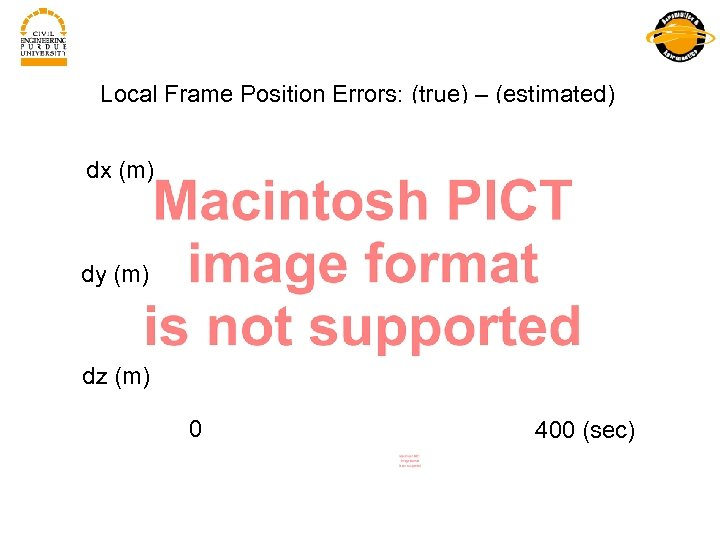Local Frame Position Errors: (true) – (estimated) dx (m) dy (m) dz (m) 0 400 (sec)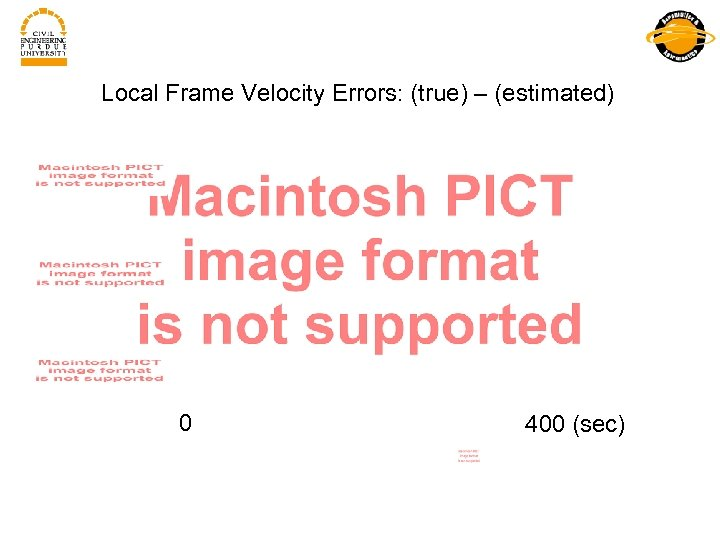Local Frame Velocity Errors: (true) – (estimated) 0 400 (sec)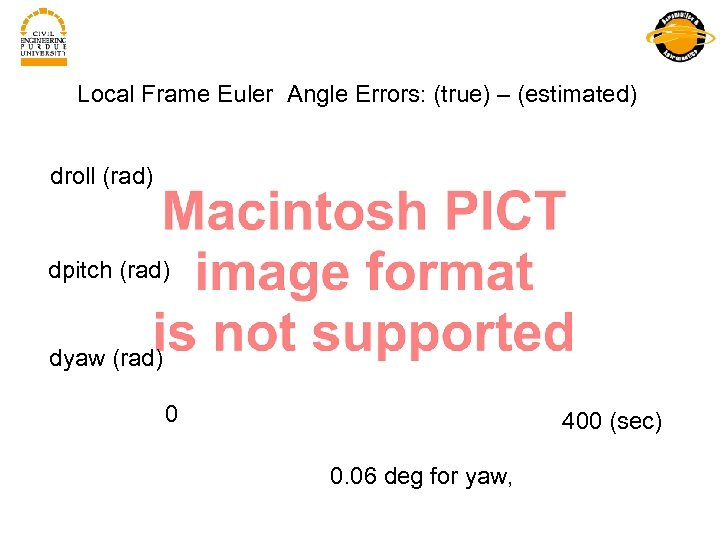Local Frame Euler Angle Errors: (true) – (estimated) droll (rad) dpitch (rad) dyaw (rad) 0 400 (sec) 0. 06 deg for yaw,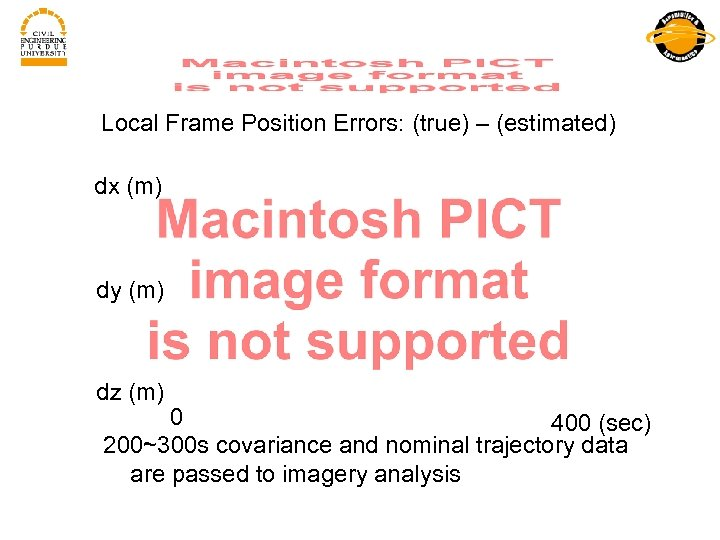Local Frame Position Errors: (true) – (estimated) dx (m) dy (m) dz (m) 0 400 (sec) 200~300 s covariance and nominal trajectory data are passed to imagery analysis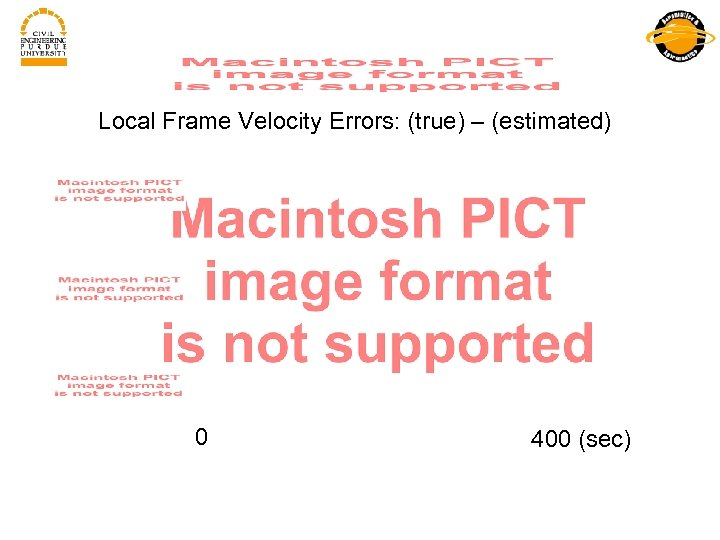Local Frame Velocity Errors: (true) – (estimated) 0 400 (sec)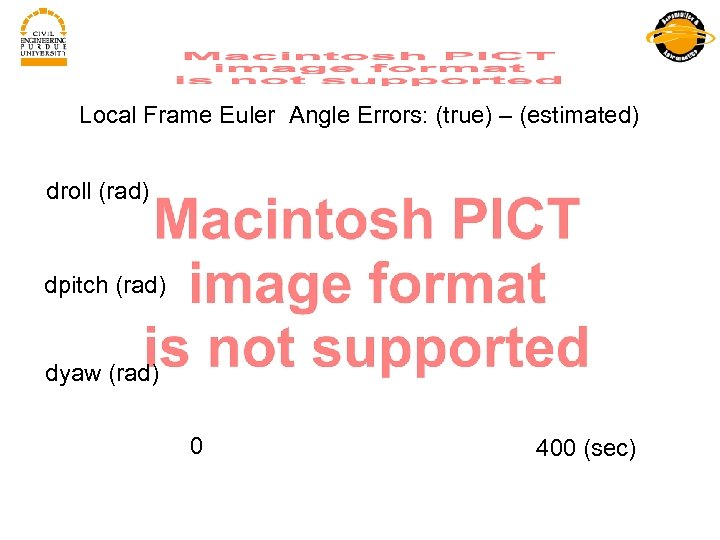Local Frame Euler Angle Errors: (true) – (estimated) droll (rad) dpitch (rad) dyaw (rad) 0 400 (sec)